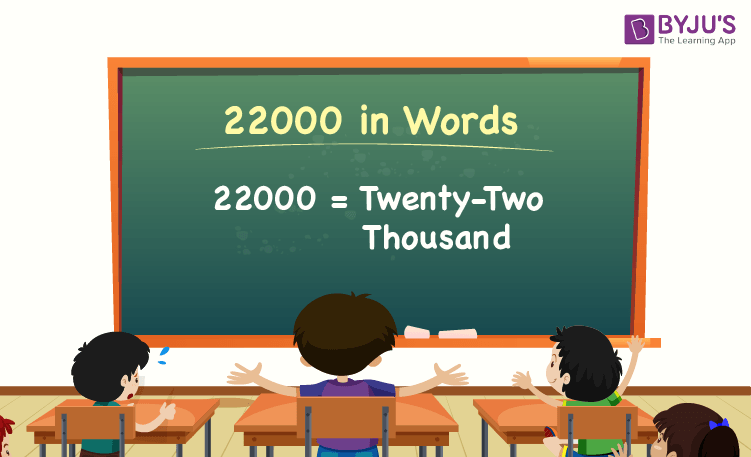# 22000 in Words

22000 in words is Twenty-two Thousand. For example, if you spent Rs. 22000 in three months, you can write, “I have spent Rs. Twenty-two thousand in three months”. The number name of 22000 can be written using the place value of a number.

 22000 in Words: Twenty-two Thousand

In this article, we will discuss how to write 22000 in words, and look at the solved examples in detail.

## How to Write 22000 in Words?To write the number name of 22000, first, determine the place value of each digit of the given number 22000. Hence, for the number 22000, the digit in one’s place is 0, the digit in ten’s place is 0, the digit in hundred’s place is 0, and the digit in thousand’s place is 22.

For the number 22000,

1’s place of 22000 is 0

10’s place of 22000 is 0.

100’s place of 22000 is 0.

1000’s place of 22000 is 22.

Thus, the place value chart for 22000 is:

 Thousands Hundreds Tens Ones 22 0 0 0

Therefore, the number 22000 in words is Twenty-two Thousand.

### Examples

Example 1:

Find the value of 18000 + 4000. Describe the value in words.

Solution:

Given expression: 18000 + 4000

⇒ 18000 + 4000 = 22000

So, the value of 18000 + 4000 is 22000.

Hence, 22000 in words is twenty-two thousand.

Example 2:

Express the value of twenty-two thousand minus two thousand in words.

Solution:

Twenty-two thousand = 22000

Two thousand = 2000

Twenty-two thousand minus two thousand = 22000 – 2000 = 20000

Hence, the value of twenty-two thousand minus two thousand is twenty thousand in words.

## Frequently Asked Questions on 22000 in Words

Q1

### Write 22000 in words.

22000 in words is twenty-two thousand.

Q2

### Express the value of 21000 + 1000 in words.

Simplifying 21000 + 1000, we get 22000. Hence, 22000 in words is twenty-two thousand.

Q3

### How to write twenty-two thousand in numbers?

Twenty-two thousand in numbers is 22000.

Q4

### Is the number 22000 an even number?

Yes, the number 22000 is an even number.

Q5

### Is 22000 a perfect square number?

No, 22000 is not a perfect square number.